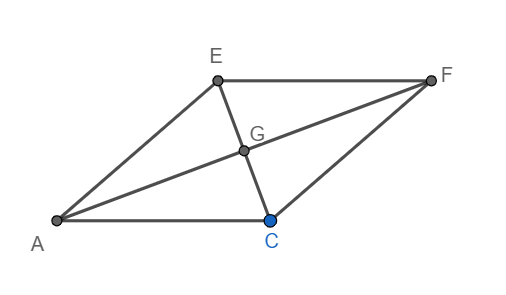QuestionAnswers

# ACEF is a rhombus with AC = 20cm and EC = 28 cm. The length of AF is :[a] $\sqrt{204}$ cm[b] 204 cm[c] $2\sqrt{204}$ cm[d] 408 cm

Hint: Use the property that the diagonals of a rhombus bisect each other at right angles. Hence, use Pythagoras theorem to find the length of the diagonal. Alternatively, find the area of rhombus using heron’s formula in triangle ECF and using the property that a diagonal of a parallelogram divides the parallelogram into two congruent triangles and find the area again using the area of a rhombus $=\dfrac{1}{2}{{d}_{1}}{{d}_{2}}$. Hence compare the two areas and from a linear equation in ${{d}_{2}}$. Solve for ${{d}_{2}}$. The value of ${{d}_{2}}$ gives the length of the second diagonal.

We know that the diagonal of a rhombus bisect each other at right angles. Hence we have an angle EGF is a right angle.
Now, given that EC = 28 cm.
Since G is the midpoint of EC, we have EG = 14 cm.
Also EF = 20cm.
We know that in a right-angled triangle, the sum of the squares of legs is equal to the square of the hypotenuse {Pythagoras theorem}.
Using Pythagoras theorem in triangle EGF, we have
$\text{E}{{\text{G}}^{2}}+\text{G}{{\text{F}}^{2}}=\text{E}{{\text{F}}^{2}}$
Substituting EG = 14 and EF = 20, we get
\begin{align} & {{14}^{2}}+\text{G}{{\text{F}}^{2}}={{20}^{2}} \\ & \Rightarrow 196+\text{G}{{\text{F}}^{2}}=400 \\ \end{align}
Subtracting 196 from both sides, we get
$\text{G}{{\text{F}}^{2}}=400-196=204$
Taking square root on both sides, we get
$\text{GF = }\sqrt{204}$ cm
Since G is the midpoint of AF, we have
$\text{AF=2GF=2}\sqrt{204}$ cm.
Hence option [c] is correct.

Note: Alternative Solution:
We have in triangle ECF, EC = 28cm, EF = 20cm and CF = 20cm.
Hence we have a =28, b = 20 and c = 20.
Hence, we have s $=\dfrac{a+b+c}{2}=\dfrac{28+20+20}{2}=34$
We know from heron’s formula that area of a triangle $=\sqrt{s\left( s-a \right)\left( s-b \right)\left( s-c \right)}$
Using heron's formula for a = 28, b= 20, c= 20 and s =34
We have the area of triangle ECF $=\sqrt{34\left( 34-28 \right)\left( 34-20 \right)\left( 34-20 \right)}=28\sqrt{51}$.
Since the diagonal of a parallelogram divides the parallelogram into two congruent triangles, we have
$ar\left( \Delta \text{ECF} \right)=ar\left( \Delta \text{ECA} \right)$
Hence area of the rhombus ACFE $=ar\left( \Delta \text{ECF} \right)+ar\left( \Delta \text{ECA} \right)=28\sqrt{51}+28\sqrt{51}=56\sqrt{51}$
Also area of a rhombus $=\dfrac{1}{2}{{d}_{1}}{{d}_{2}}$
We have ${{d}_{1}}=28.$
Hence, we have
\begin{align} & \dfrac{1}{2}\left( 28 \right){{d}_{2}}=56\sqrt{51} \\ & \Rightarrow {{d}_{2}}=4\sqrt{51}=2\sqrt{204} \\ \end{align}
Hence the length of the other diagonal is $2\sqrt{204}$